Symmetry (Maths) Class 7 - NCERT Questions
Q 1.

Copy the figures with punched holes and find the axes of symmetry for the following :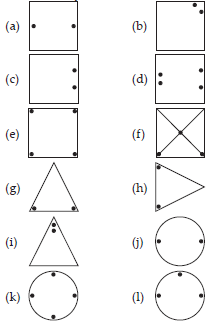SOLUTION: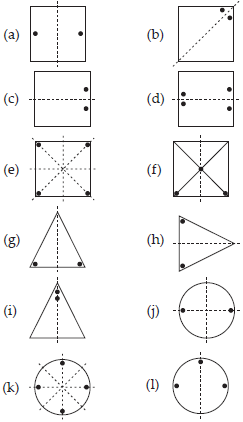Q 2.

Given the line(s) of symmetry, find the other hole(s).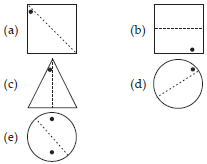SOLUTION: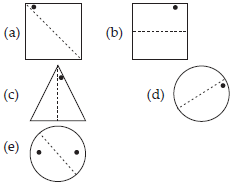Q 3.

In the following figures, the mirror line (i.e., the line of symmetry) is given as a dotted line. Complete each figure performing reflection in the dotted (mirror) line. (You might perhaps place a mirror along the dotted line and look into the mirror for the image). Are you able to recall the name of the figure you complete?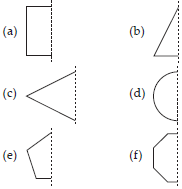SOLUTION: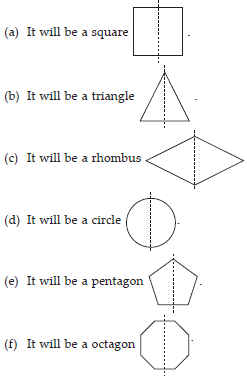Q 4.

The following figures have more than one line of symmetry. Such figures are said to have multiple lines of symmetry.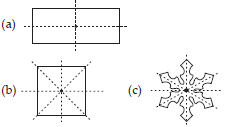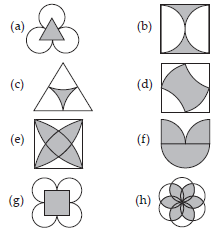SOLUTION: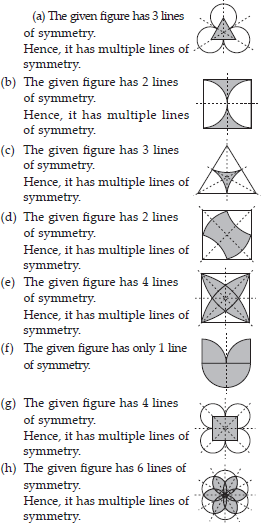Q 5.

Copy the figure given here.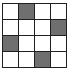Take any one diagonal as a line of symmetry and shade a few more squares to make the figure symmetric about a diagonal. Is there more than one way to do that? Will the figure be symmetric about both the diagonals?

SOLUTION:

We can shade a few more squares so as to make the given figure symmetric about any of its diagonals.
Yes, the figure is symmetric about both the diagonals. There is more than one way so as to make the figure symmetric about a diagonal as we can choose any of its 2 diagonals.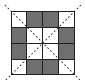Q 6.

Copy the diagram and complete each shape to be symmetric about the mirror line(s).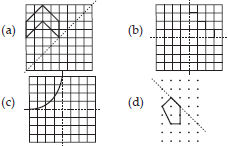SOLUTION: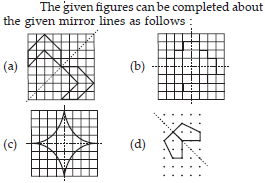Q 7.

State the number of lines of symmetry for the following figures.
(A) An equilateral triangle
(B) An isosceles triangle
(C) A scalene triangle (D) A square
(E) A rectangle (F) A rhombus
(G) A parallelogram (H) A quadrilateral
(I) A regular hexagon (J) A circle

SOLUTION: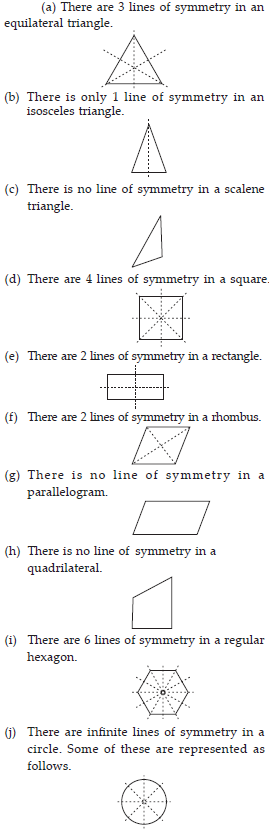Q 8.

What letters of the English alphabet have reflectional symmetry (i.e.,
symmetry related to mirror reflection) about. (A) a vertical mirror
(B) a horizontal mirror
(C) both horizontal and vertical mirrors

SOLUTION: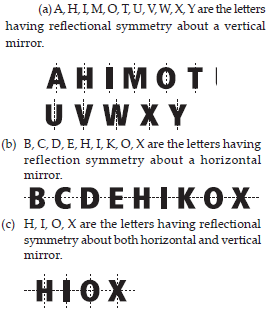Q 9.

Give three examples of shapes with no line of symmetry.

SOLUTION: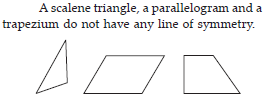Q 10.

What other name can you give to the line of symmetry of
(A) an isosceles triangle?
(B) a circle?

SOLUTION: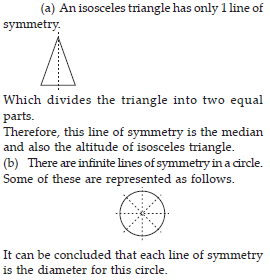Q 11.

Which of the following figures have rotational symmetry of order more than 1 :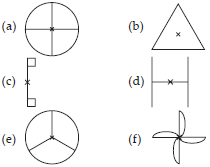SOLUTION: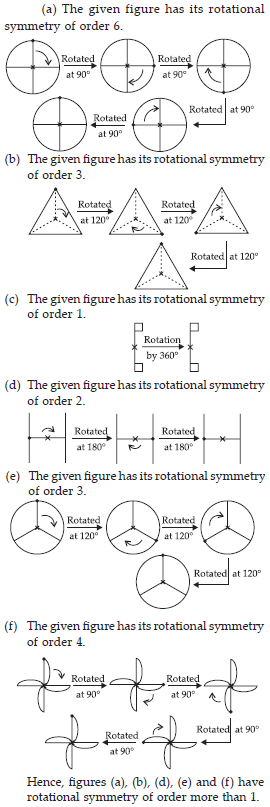Q 12.

Give the order of rotational symmetry for each figure.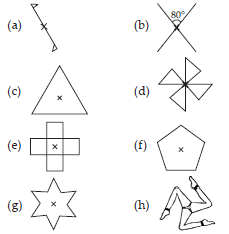SOLUTION: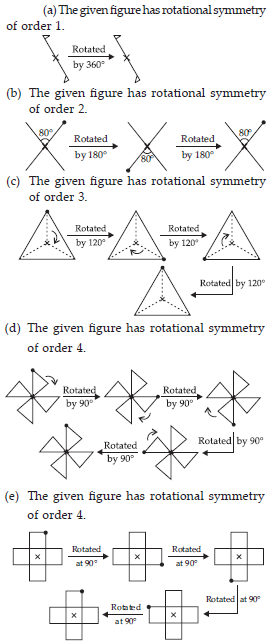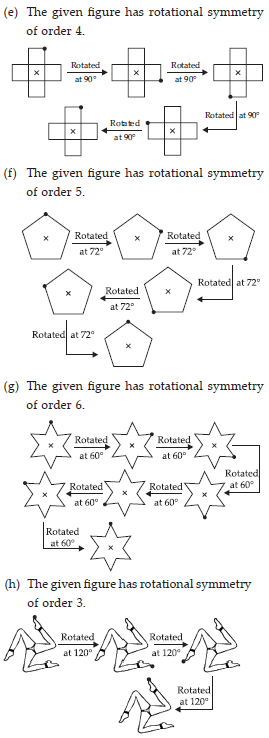Q 13.

Name any two figures have both line symmetry and rotational symmetry.

SOLUTION:

Equilateral triangle and regular hexagon have both line symmetry and rotational symmetry.

Q 14.

Draw, whether possible, a rough sketch of
(i) a triangle with both line and rotational symmetries of order more than 1.
(ii) a triangle with only line symmetry and no rotational symmetry of order more than 1.
(iii) a quadrilateral with a rotational symmetry of order more than 1 but not a line symmetry.
(iv) a quadrilateral with line symmetry but not a rotational symmetry of order more than 1.

SOLUTION: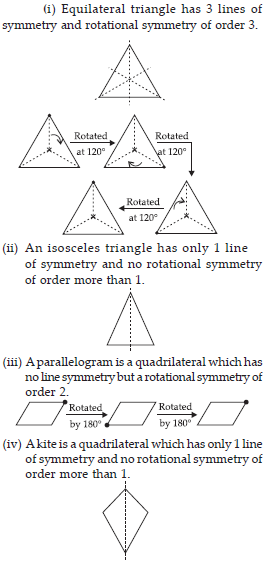Q 15.

If a figure has two or more lines of symmetry, should it have rotational symmetry of order more than 1?

SOLUTION:

Yes, if a figure has two or more lines of symmetry, then it will definitely have its rotational symmetry of order more than 1.

Q 16.

Fill in the blanks :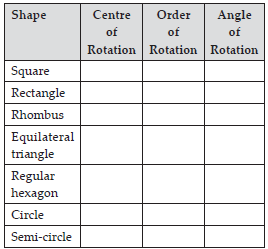SOLUTION: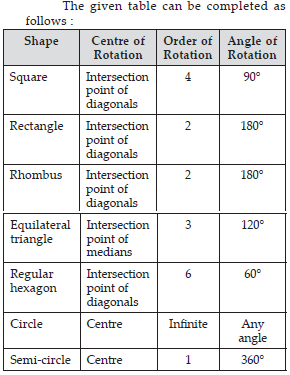Q 17.

Name the quadrilaterals which have both line and rotational symmetry of order more than 1.

SOLUTION:

Square, rectangle, and rhombus are the quadrilaterals which have both line and rotational symmetry of order more than 1. A square has 4 lines of symmetry and rotational symmetry of order 4. A rectangle has 2 lines of symmetry and rotational symmetry of order 2. A rhombus has 2 lines of symmetry and rotational symmetry of order 2.

Q 18.

After rotating by 60° about a centre, a figure looks exactly the same as its original position. At what other angles will this happen for the figure?

SOLUTION:

It can be observed that if a figure looks symmetrical on rotating by 60°, then it will also look symmetrical on rotating by 120°, 180°, 240°, 300°, 360° i.e., further multiples of 60°.

Q 19.

Can we have a rotational symmetry of order more than 1 whose angle of rotation is (i) 45°? (ii) 17°?

SOLUTION:

It can be observed that if the angle of rotation of a figure is a factor of 360°, then it will have a rotational symmetry of order more than 1.
(i) It can be checked that 45° is a factor of 360°. Therefore, the figure having its angle of rotation as 45° will have its rotational symmetry of order more than 1.
(ii) 17° is not a factor of 360°. Therefore, the figure having its angle of rotation as 17° will not be having its rotational symmetry of order more than 1.